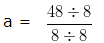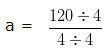# Linear Equations Worksheet for Class 6

Please check Solutions of Linear Equations Worksheet for Class 6 at the end of the questions.

Download Linear Equations Worksheet for Class 61. If a number triple itself and the result is increased by 5 we get 35. Find the number?
a) 8
b) 12
c) 10

2. If a number double itself and the result is increased by 4 we get 34. Find the number?
a) 14
b) 15
c) 13

3. Find the value of “a” if,
2a + 9 = 57 – 6a
a) 7
b) 6
c) 8

4. 6 times a number is 42
a) 6 + a = 42
b) 6a = 42
c) 6 + 42 = a

5. Verify by substitution, whether one of the root of the equation 5a – 7 = 23 is true, when a = 6
a) True
b) False

6. Find the Value of “a” if, (4a+8)/6 = 4
a) 4
b) 6
c) 5

7. If a bag contains 25 paisa and 50 paisa coins whose total values is Rs 25 and if the number of 25 paisa coins is two times that of 50 paisa coins, find the number of each type of coins ?
a) 15, 60
b) 35, 40
c) 25, 50

8. Solve the given equation for the value of a?
4a/5 = 24
a) 32
b) 30
c) 27

9. If Samiksha is 12 years older than Kanchan and the sum of their age is 36 years, what are their present ages ?
a) 12 , 24
b) 11 , 25
c) 13 , 23

### Linear Equations Worksheet for Class 6 Solutions

Linear Equations Worksheet for Class 6 – Solution 1

Let the required number be x
If the Number is triple itself, we get 3 × x
We know that,
3 × x + 5 = 35
Transposing + 5 to -5 (Addition Changes to Subtraction)
3x = 35 – 5
3x = 30
x =30/3
x = 10
The required number is x = 10

Correct Answer – c) 10

Linear Equations Worksheet for Class 6 – Solution 2

Let the required number be x
If the Number is double itself, we get 2 × x
We know that,
2 × x + 4 = 34
Transposing + 4 to -4 (Addition Changes to Subtraction)
2x = 34 – 4
2x = 30
x = 30/2
x = 15
The required number is x = 15

Correct Answer – b) 15

Linear Equations Worksheet for Class 6 – Solution 3

Given Equation:
2a + 9 = 57 – 6a
In the given expression, write variables on one side and Exponents on other side
Therefore,
2a + 9 = 57 – 6a
Transposing + 9 to -9
2a + 6a = 57 – 9
Take “a” as common and add the variables
( 2 + 6 )a = 48
8a = 48
a = 48/8
Divide Numerator and Denominator with their HCF i.e, 8a = 6/1
a = 6
Hence, the value of “a” is 6

Correct Answer – b) 6

Linear Equations Worksheet for Class 6 – Solution 4

Suppose, the required number is ‘ a ‘
So, 6 times the number will be 6a
Therefore, 6a = 42
Hence, the Required Equation is
6a = 42

Correct Answer – b) 6a = 42

Linear Equations Worksheet for Class 6 – Solution 5

Given Equation :
5a – 7 = 23
Now, replace the value of a = 6 in the given equation
On substituion, the given equation becomes 5 x 6 – 7 = 23
According to BODMAS Rule, we will do multiplication first, i.e 5 x 6 = 30
Therefore, the equation would now be 30 – 7 = 23 or 23 = 23
LHS = RHS = 23
Hence, a = 6 verified.

Correct Answer – a) True

Linear Equations Worksheet for Class 6 – Solution 6

(4a+8)/6 = 4
On Cross Multiplying,
4a + 8 = 4 x 6
4a + 8 = 24
Transposing + 8 to -8 (Addition Changes to Subtraction)
4a = 24 – 8
4a = 16
On Cross Multiplying
a = 16/4
a = 16/4 = 4/1 = 4
Value of a = 4

Correct Answer – a) 4

Linear Equations Worksheet for Class 6 – Solution 7

Let number of the 50 paisa coins be x
As 25 paisa coins are 2 times of 50 paisa coins
Therefore, the number of 25 paisa coins will be ( 2 × x )
Now, we are given that
50 × x + 25 × ( 2 × x ) = 2500
Both the expressions have same Variable so we can add them,
or 50 × x + 50 × x = 2500
Take x as common
( 50 + 50 )x = 2500
100x = 2500
x = 2500/100
x = 25
Therefore the number of 50 paisa coins is 25
Number of 25 paisa coins = 2 × x
Number of 25 paisa coins = 2 × 25
Number of 25 paisa coins = 50

Correct Answer – c) 25, 50

Linear Equations Worksheet for Class 6 – Solution 8

Given Equation:
4a/5 = 24
On Cross Multiplication,
4a = 24 x 5
4a = 120
Therefore,
a = 120/4
Dividing both the Numerator and Denominator by their HCF i.e, 4= 30/1 = 30
So, the value of ‘a’ = 30

Correct Answer – b) 30

Linear Equations Worksheet for Class 6 – Solution 9

Let the present age of Kanchan be 1a
Since Samiksha is 12 years older than Kanchan
Present age of Samiksha will be ( 1a + 12 ) years
Given,
Sum of their ages = 36
i.e,
1a + ( 1a + 12 ) = 36
Take “a” as common
( 1 + 1 )a + 12 = 36
2a + 12 = 36
Transposing + 12 to -12 (Addition Changes to Subtraction)
2a = 36 – 12
2a = 24
or in other words
a = 24/2
a = 12
Present age of Kanchan = 12 Years
Present age of Samiksha = (1 x a + 12) years
= (1 x 12 + 12) years
= 24 years

Correct Answer – a) 12 , 24

### 2 thoughts on “Linear Equations Worksheet for Class 6”

1.It was helpful. Keep on posting!

Reply
2.The sums are easy try to make them hard.

Reply